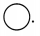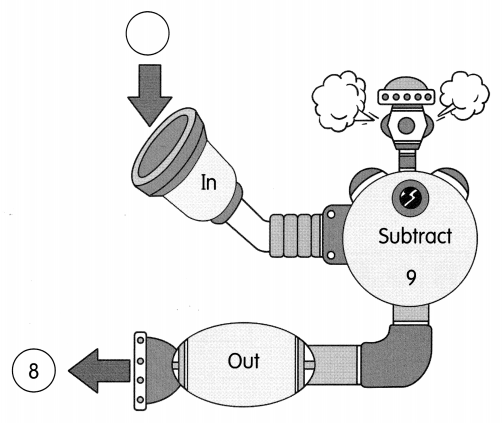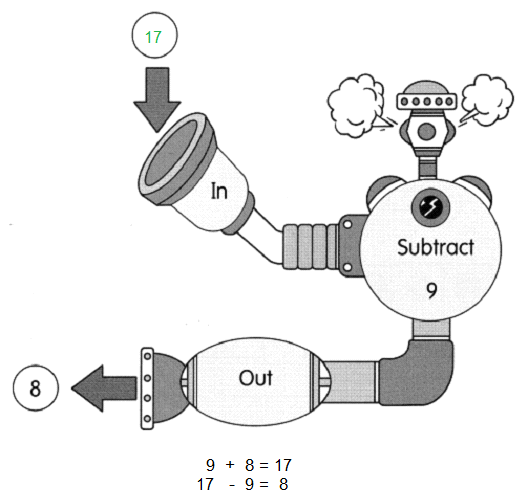# Math in Focus Grade 1 Chapter 8 Practice 4 Answer Key Ways to Subtract

Practice the problems of Math in Focus Grade 1 Workbook Answer Key Chapter 8 Practice 4 Ways to Subtract to score better marks in the exam.

## Math in Focus Grade 1 Chapter 8 Practice 4 Answer Key Ways to Subtract

Group the numbers into a 10 and ones. Then subtract.

Example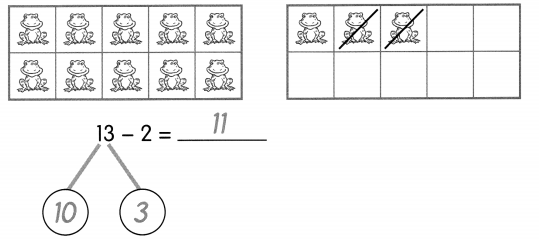Question 1.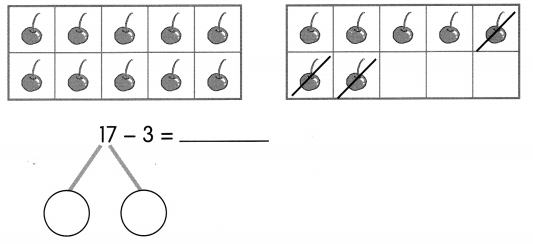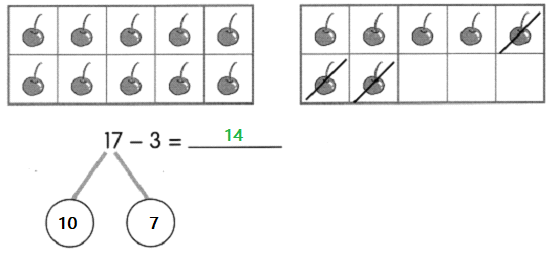Question 2.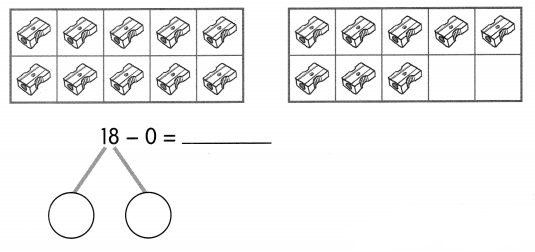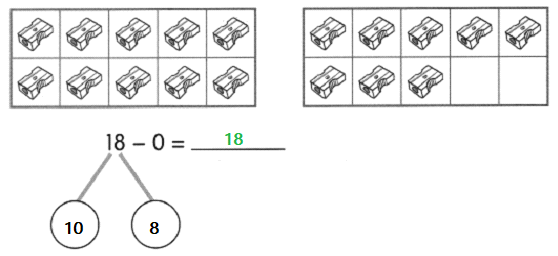Question 3.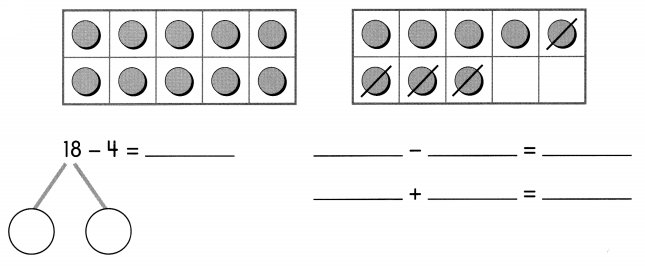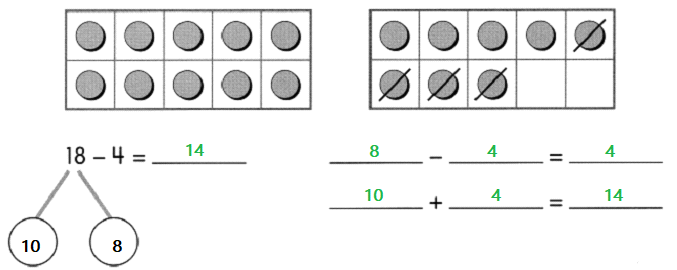Question 4.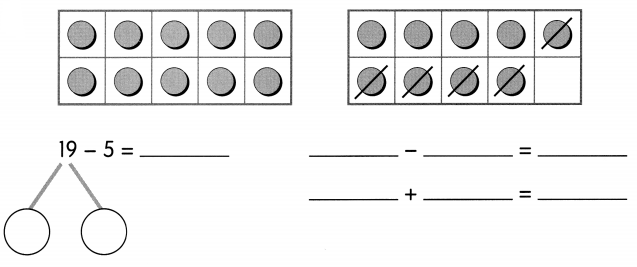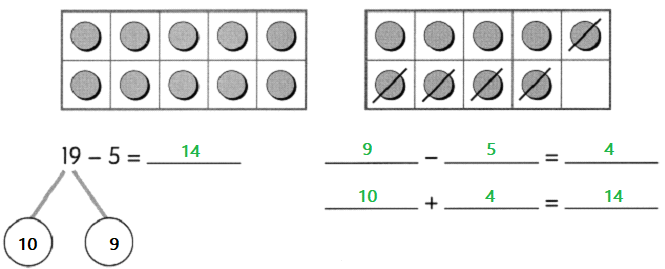Question 5.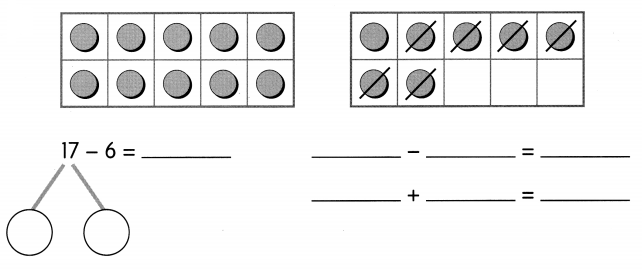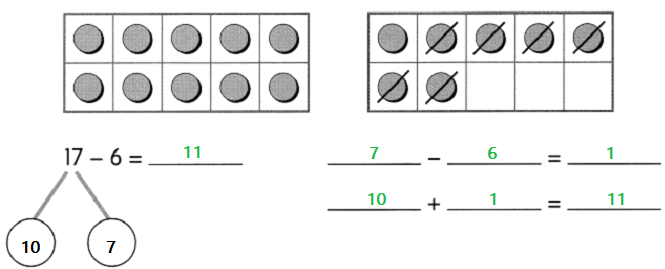Group the numbers into a 10 and ones. Then subtract.

Example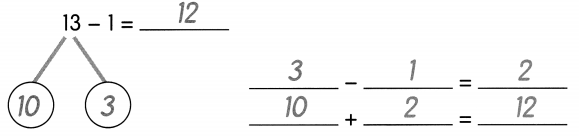Question 6.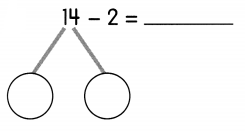_____ – ____ = _____
_____ + ____ = _____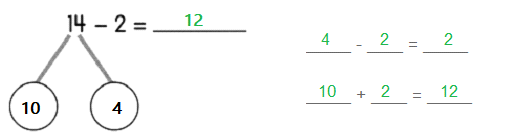Question 7.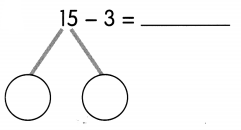_____ – ____ = _____
_____ + ____ = _____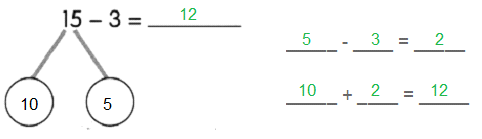Question 8.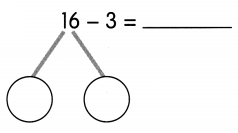_____ – ____ = _____
_____ + ____ = _____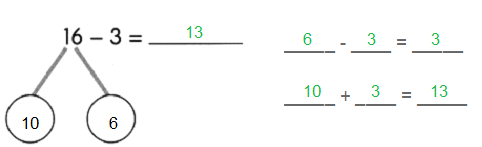Question 11.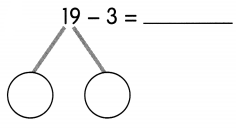_____ – ____ = _____
_____ + ____ = _____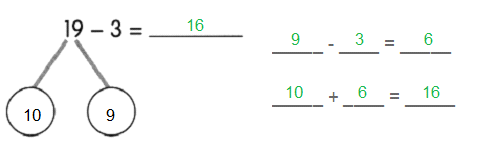Group the numbers into a 10 and ones. Then subtract.

Example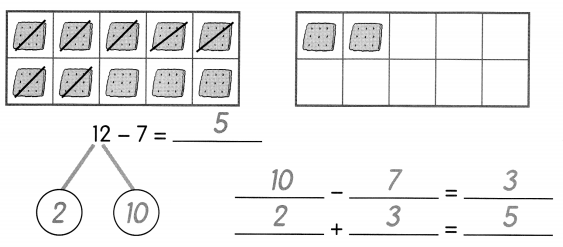Question 10.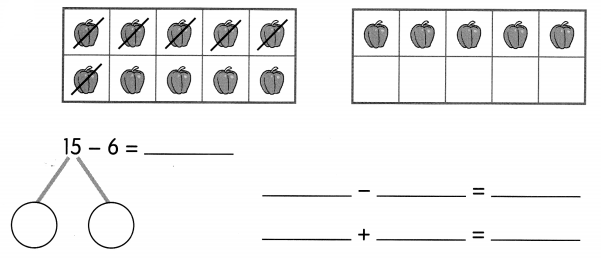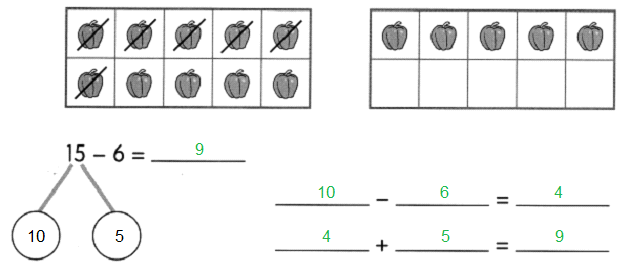Question 11.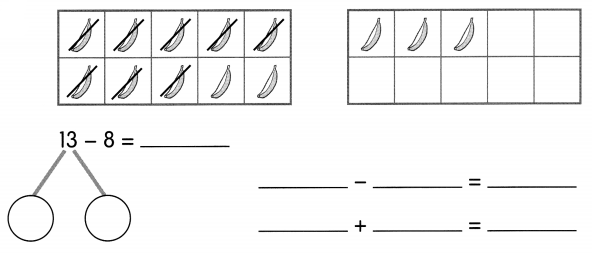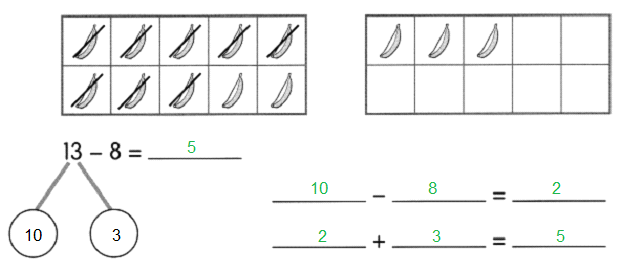Question 12.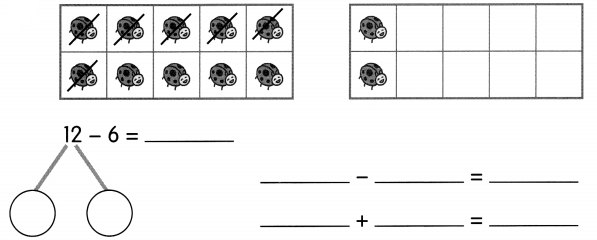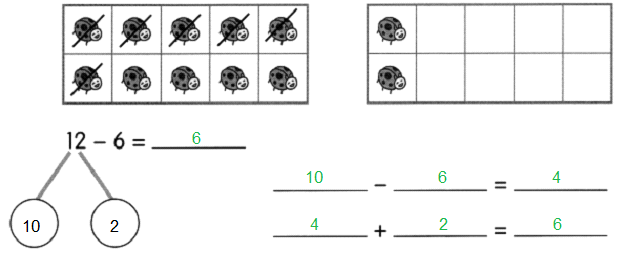Question 13.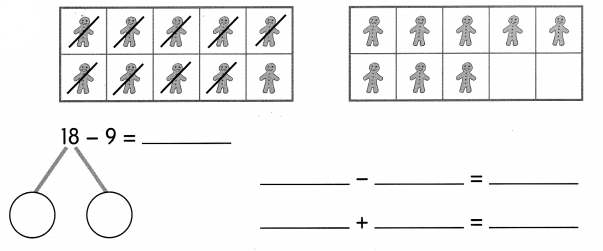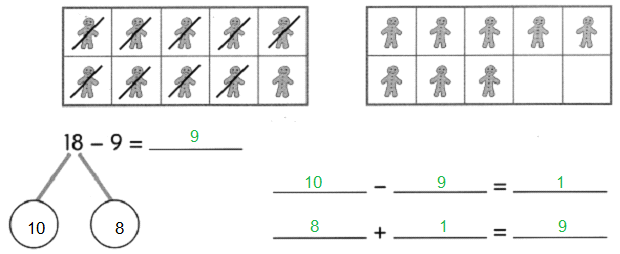Complete each subtraction sentence.

Question 14.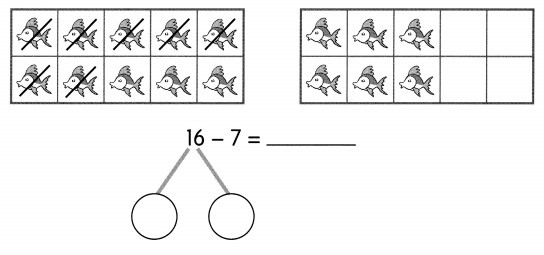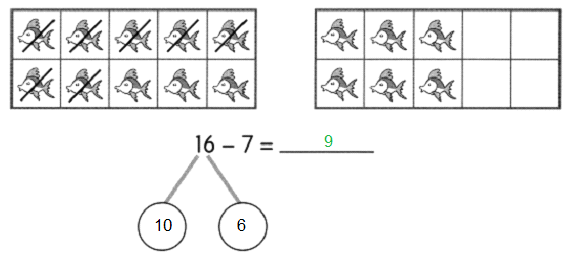Question 15.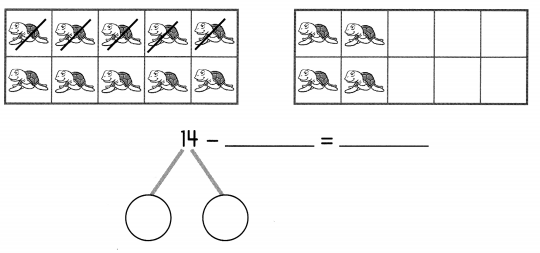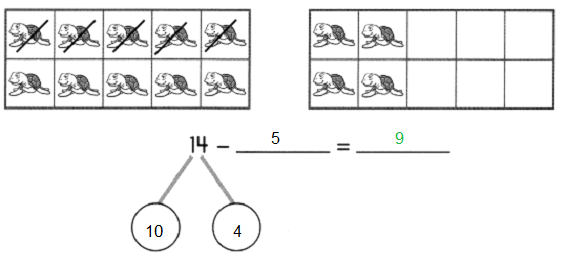Question 16.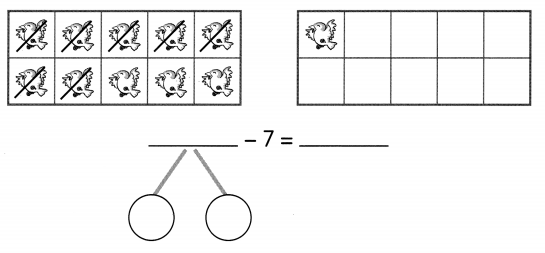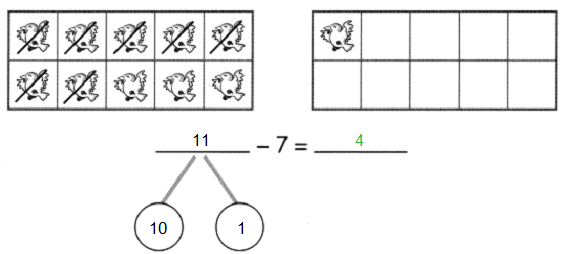Complete each subtraction sentence.

Question 17.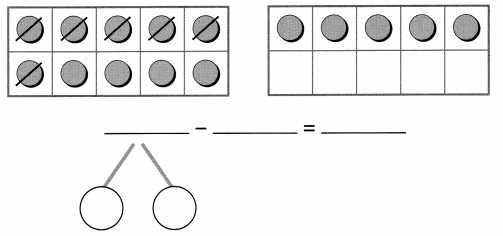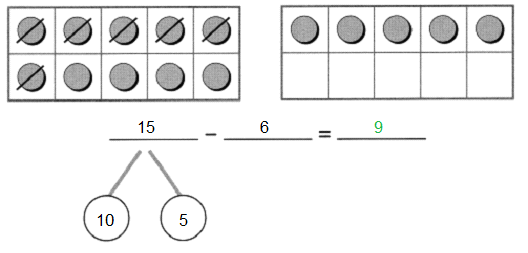Question 18.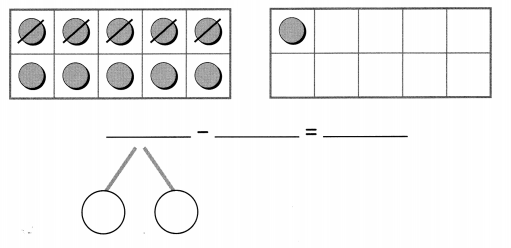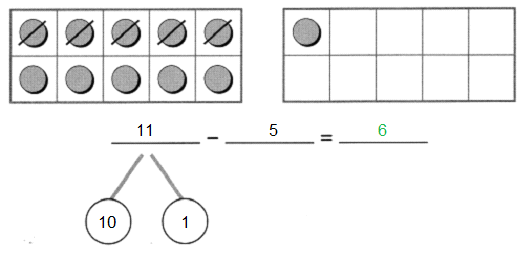Question 19.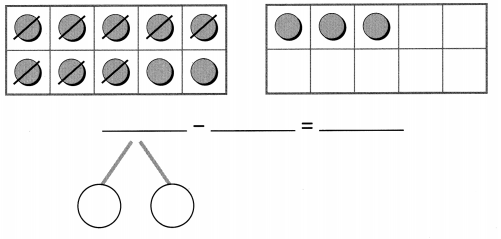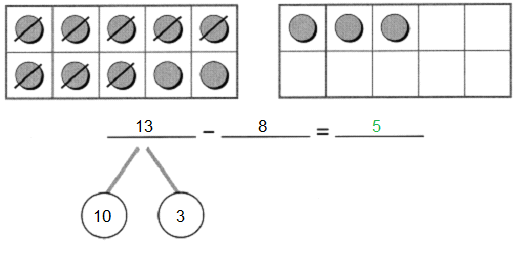Complete the number bonds. Subtract.

Question 20.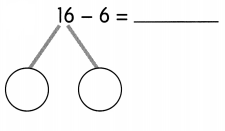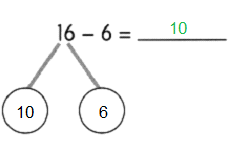Question 21.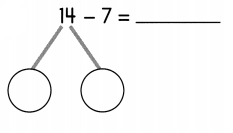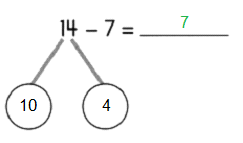Which number fell into the number machine? Write the number in